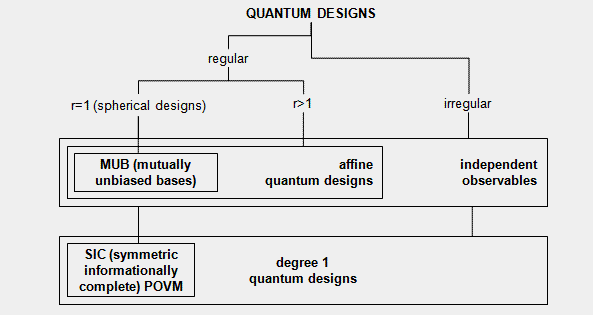Gerhard Zauner

# Introduction

Quantum Theory lives in complex Hilbert Spaces. The closed linear subspaces of seperable Hilbert Spaces form a so called orthocomplemented and quasimodular lattice, featuring - in opposition to classical (boolean) logic - an own "Logic of Quantum Mechanics" (G.Birkhoff, J.v.Neumann, 1936). These subspaces also replace the sigma-algebra of classical probability theory with probability measures on it fixed via the Born Rule, resp. Gleason's theorem.
Allthough most of (current) quantum theory uses infinite dimensional (often even non-seperable) Hilbert Spaces, interesting features of this logic and probability theory already appear in finite dimensions (e.g. entanglement, Bell's inequality, GHZ states, the Kochen-Specker theorem, the algorithms of quantum computing, etc.).

(Closed) linear subspaces can be described by orthogonal projection operators P (= P2 = P*). Quantum designs are sets of orthogonal projection matrices in d-dimensional Hilbert Spaces, with certain features: A fundamental differentiation is whether the quantum design is regular, this means that all assigned subspaces have same dimension r, and here the special case r=1 (this means that the subspaces are spanned by single (unit) vectors).

Here is an overview of the most important special cases (as explained on the next pages):Dieser Text ist nur auf Englisch verfügbar